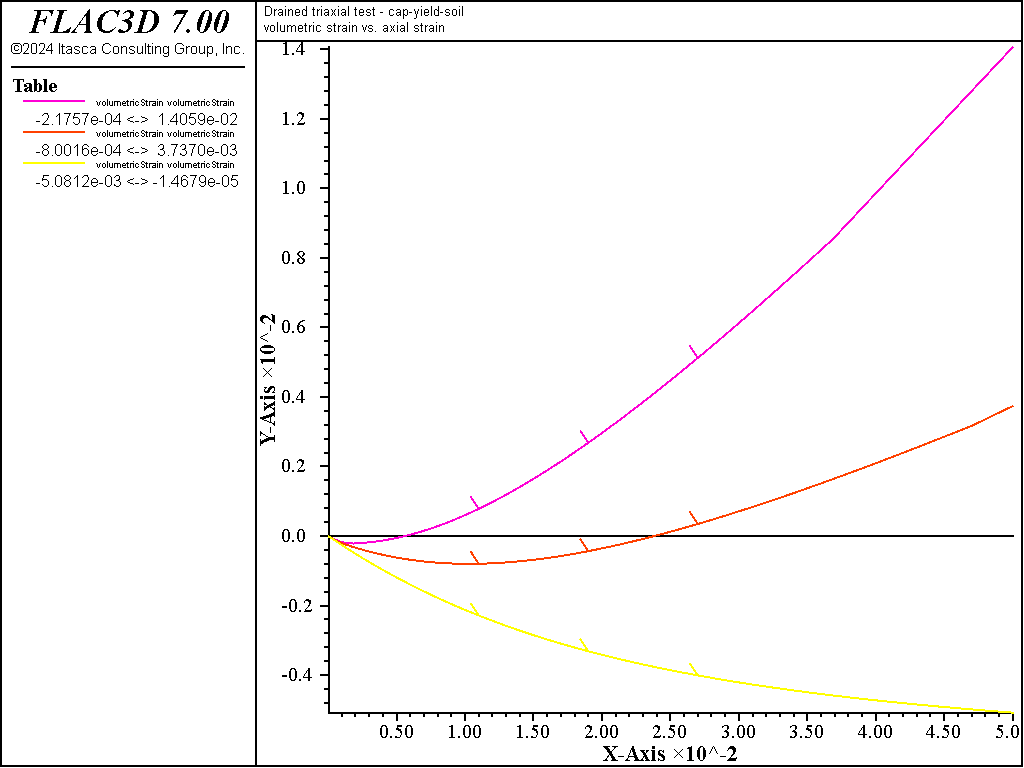FLAC3D Theory and Background • Constitutive Models

# Drained Triaxial Test with CYSoil Model — Constant Dilation

Note

To view this project in FLAC3D, use the menu command Help ► Examples…. Choose “ConstitutiveModels/ DrainedTriaxialConstantDilationCYSoil” and select “DrainedTriaxialConstantDilationCYSoil.prj” to load. The project’s main data file is shown at the end of this example.

Triaxial tests on dense, medium, and loose sand are simulated using the CYSoil model. The model properties are listed in Table 1. This example does not consider yielding on the cap; therefore, flag_cap is set to 0 (default value). In this case, initial pressure, $$p_{ini}$$, is required; it is used by the code to calculate for bulk and shear moduli. The dilation is constant; therefore, flag_dilation is set to 1, and a value is assigned to the dilation property. The initial stress state is isotropic, with mean pressure equal to 100 kPa. The cell pressure is kept constant during the test, the base of the model is fixed in the axial ($$y-$$) direction, and an axial velocity of 10-6 m/step is applied at the top of the model. In addition, three unloading/reloading excursions are performed.

Table 1: CYSoil Model Properties
Parameter Dense Medium Loose
$$G_{ref}$$ 300 225 150
$$\phi_f$$ 40 35 30
$$\psi_f$$ 10 5 0
$$c$$   0 or 100 kPa
$$p_{ref}$$ (kPa)   100
$$p_c$$ (kPa)   100
$$\nu$$   0.2
$$m$$   0.5
$$R_f$$   0.9
$$\beta$$   0.35

A plot of deviatoric stress versus axial strain for the cohesionless simulation is shown in Figure 1 for dense, medium, and loose sand cases. The plot shows the hyperbolic behavior expected from the model, and the higher failure level achieved by the denser soil. Three unloading/reloading excursions are also shown in the figure. The plot of volumetric strain versus axial strain for the cohesionless simulation is shown in Figure 2. The volumetric behavior is monotonic in the plot; the dilatant behavior of the dense sand is clearly shown. The comparable results for the simulation with cohesion set to 100 kPa are shown in Figure 3 and Figure 4. It is seen from Figure 3 that the initial stiffness of cohesive sand is higher than that of the sand without cohesion. Similarly, deviatoric stress at failure is higher for the cohesive material.

breakFigure 1: $$\mid \sigma_1 - \sigma_3 \mid$$ (in kPa) versus axial strain for dense, medium, and loose sand—constant dilation.Figure 2: Volumetric strain versus axial strain for dense, medium, and loose sand—constant dilation.Figure 3: $$\mid \sigma_1 - \sigma_3 \mid$$ (in kPa) versus axial strain for dense, medium, and loose sand—constant dilation (dilation = 100 kPa).Figure 4: Volumetric strain versus axial strain for dense, medium, and loose sand—constant dilation (dilation = 100 kPa).

Data File

DrainedTriaxialConstantDilationCYSoil.dat

model large-strain off
; Drained Triaxial Tests - Constant Dilation
model title "Drained triaxial test - cap-yield-soil"
;
zone create brick size 1 1 1
zone face skin
zone cmodel assign cap-yield
;
zone initialize stress xx -100. yy -100. zz -100.
zone face apply stress-norm -100. range group 'East' or 'West'
zone face apply stress-norm -100. range group 'North' or 'South'
zone face apply velocity-z     0. range group 'Bottom'
zone face apply velocity-z -1e-6  range group 'Top'
;
zone property density 1000 pressure-reference=100. exponent=1.0 ...
beta=0.35 poisson 0.2
zone property flag-dilation=1 friction-mobilized=0
zone property shear-reference=[_Gref] friction=[_friu] ...
cohesion=[_coh] dilation=[_dilu]
zone property flag-cap=0 pressure-initial=100.
;
fish define _cy_q
global _cy_q = zone.prop(_z, 'stress-deviatoric')
global _vsi  = zone.strain.vol.inc(_z)
end
;
history interval 50
zone history displacement-z position (1,1,1)
fish history _cy_q
fish history _vsi
;
fish define trip
loop local i (1,3)
command
zone face apply velocity-z -1e-6 range group 'Top'
model step 8000
zone face apply velocity-z  1e-7 range group 'Top'
mode step 6000
zone face apply velocity-z -1e-7 range group 'Top'
model step 6000
end_command
end_loop
end
;
model step 3000
[trip]
zone face apply velocity-z -1e-6  range group 'Top'
model step 23000
;
history export '2' vs '1' reverse table 'deviatoricStress'
history export '3' vs '1' reverse table 'volumetricStrain'
table 'deviatoricStress' ...
export [string.build('deviatoricStress%1', _IChoice)] truncate
table 'volumetricStrain' ...
export [string.build('volumetricStrain%1', _iChoice)] truncate
;